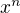First we define some terms:

## Algebraic Structure (Grouppoid)

A Algebraic Structure (Gruppoid) (G,op) is a set G together with a binary operation op, where op : G x G -> G . G has to be closed over by op (op cannot be used to leave G)

## Halfgroup

A Halfgroup is a Grouppoid where op is associative, that is: ((x op y) op z) = (x op (y op z))

## Monoid

A Monoid is a Halfgroup where a Neutral Element e exists so that (e op x) = (x op e) = x for all a ∈ G

## Group

A Group is a Monoid where any element x has (one) Inverse Elementso that.

## Commutative Group

A Commotiative Group (Abelian Group) is a Group where op is always commutative.

## Power of Elements of the Group

The nth Power of x (where n is a whole number), written, is defined as:Then, the laws of power holds:((+) and (⋅) are operations on the whole numbers)

### Group Homomorphism

A map φ : G -> H between (the sets of) groups (G,⨯) and (H,%) is called Group Homomorphism iff ∀a∈G ∀b∈G:### Group Isomorphism

A Group Isomorphism is a Group Homomorphism where φ is also a bijection. The Groups G and H then are called isomorph, written (G ~= H).

### Kernel

The Kernel of φ, written ker φ, where φ is a Group Homomorphism, is defined as:(whereis the neutral element of H).

## Ring

A Ring is an Algebraic Structure (G,+,⋅) over a set G with two binary operations (+) and (⋅), such that all of the following hold:

• (G,+) is a Commutative Group.
• (G,⋅) is (at least) a Halfgroup.
• Distributivity holds:and## Commutative Ring

A Ring (G,+,⋅) that is commutative with respect to (⋅) is called Commutative Ring.

## Integrity Ring

A Commutative Ring with an element "1" without divisors of zero is called Integrity Ring. There, one can cancel out common factors (the common factors are called units (as opposed to unity!)) in equations.

## Field

A Commutative Ring with an element "1" (which is not the same as "0"), where each element except 0 is a unit (can cancel out any common factor except 0), is called Field.

## Lie Rotation Groups

• SO(n): Group of rotations in n real dimensions.
• SU(n): Group of rotations in n complex dimensions.
• SP(n): Group of rotations in n Quaternion dimensions.

## Subgroup

If G is a group, H is a subset of G and let g be an element of G, then:

H is a Subgroup if H=g⋅H⋅g^{-1} (H is preserved under conjugation).

## Conjugationwhereis the inverse.

The conjugate is a measure of how much the operation commutes.## Funny Symbols

• a H={ah: h∈H}: left coset of a.
• |G:H|: number of left cosets of H in G.
• ⋉ left semidirect product.
• ⋊ right semidirect product.
• ⋈ bicrossed product.
• orb(s): Orbit: set of elements that can be reached.
• stab(s): Stabilizer: set of elements that don't move s.